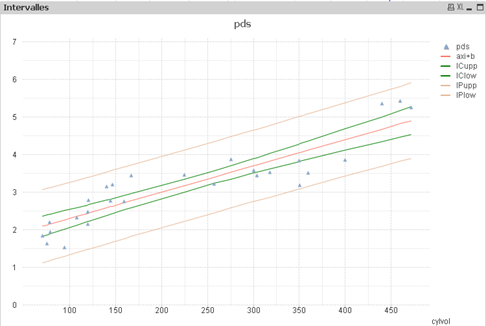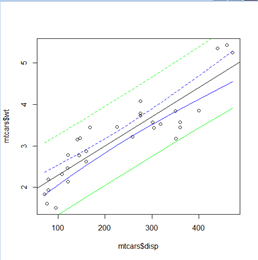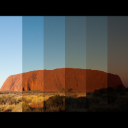# QlikView App Dev

Discussion Board for collaboration related to QlikView App Development.

Announcements
cancel
Showing results for
Did you mean:Not applicable

## calculating confidence intervals

Hi ,

I would like to generate confidence intervals for a dataset... lets use student grades as the metric (range from 1-100%).

There are QV several functions that resemble the word "confidence" intervals... lower confidence interval, upper confidence interval, however, I'm not clear what the differences are for the similar functions. So I picked: TTest1_lower(Grade_N) , There are several others, i.e. ttest_conf( Group, Value ) , ttest_lower( Group, Value ), etc.

The end result is a line chart with upper and control limits overlayed. My intent was to create the two limits: "UCL" and "LCL" via the "Reference Lines" of the Chart Properties. However, this is not working.

Stephanie

8 RepliesNot applicable
Author

I don't know if this is what you are looking for - but this is the equation that we got to work:

=

0.05 + 1.96*(stdev(Range)/sqrt(PopulationSize)))

This works for 95% confidence

Hope it helpsNot applicable
Author

Hi there,

so sorry for the late reply. I am just now revisiting the statistics application that I've been working with. What is your "mean"... is it .05...is .05 the average Range? then you add and subtract 1.96*stdev(Range)/sqrt(PopulationSize) to the "mean".... I am just restating your formula to make sure I have it right?

I hope you still remember this topic?Not applicable
Author

Hi there,

As far as I can remember the range will be the measure, ie amount of grades and the population will be the amount of students.  This will give you an absolute value.  We then worked out the % interval using a formula like this:

=(0.05 + 1.96*(stdev(Range)/sqrt(count(PopulationSize)))) / Avg(Range) which gave me a average confidence in %.

I am not quite sure how the upper and lower will work in this function as we only had to get to one answer.

If I remember correctly the 0.05 is the variable for the 95%Not applicable
Author

HI ,

I am also trying to put a 95% confidence Band on the Linear regression line .. can you Share a QVW or something to accomplish this

Thanks,

SaiSpecialist II

Concerning confidence interval of a linear regression :

this depends also on hat value of each point and on t value given by ttest function :

Confidence Interval upper bond =

\$(a) * \$(x) + \$(b) +
sqrt(sum(total aggr(pow(\$(y)  - (\$(a) * \$(x)   + \$(b)),2) , \$(id))) /n) * sqrt(n/(n-2))
*
t * sqrt(pow((\$(x) - avg(total \$(x))),2)/pow(stdev(total \$(x)),2)/(n-1) + 1/n)

with y = a*x + b  your linear regression

residual value = \$(y)  - (\$(a) * \$(x)   + \$(b))

t = ttest value for desired confidence

n = sample size

id = identifying field

where :

sqrt(sum(total aggr(pow(\$(y)  - (\$(a) * \$(x)   + \$(b)),2) , \$(id))) /n) * sqrt(n/(n-2))

= corrected standard dev of residual

sqrt(pow((\$(x) - avg(total \$(x))),2)/pow(stdev(total \$(x)),2)/(n-1) + 1/n)

= hat value at point x

and :

a= correl(\$(x),\$(y))*stdev(\$(y))/stdev(\$(x))

b= avg(\$(y)) - \$(a)*avg(\$(x))

or use LINEST_ functions

Same for prediction interval (add noise = stdev residual)

Prediction Interval upper bond :

\$(a) * \$(x) + \$(b) +
sqrt(sum(total aggr(pow(\$(y)  - (\$(a) * \$(x)   + \$(b)),2) , \$(id))) /n) * sqrt(n/(n-2))
*
t * sqrt(pow((\$(x) - avg(total \$(x))),2)/pow(stdev(total \$(x)),2)/(n-1) + 1/n + 1)verified with R

predict(model,interval = "confidence") or "prediction"regards,Specialist II

Hi Olivier

This is really interesting. Would you mind sharing the qlikview application with this example?

Thank you

LorenzoSpecialist II

Hi Lorenzo,

unfortunately I've free desktop version,

but I'm happy you find this interesting,

all expressions used are above

x :name of the field x

y : name of field or an expression

a and b of linear regression are given as a formula

and prediction or confidence interval are based upon standard formula

if you have your own sample, send/attach to make together what is interesting you :

hat values, cook's distance and other residual analysis factors can be calculated within Qlik without calling R.

regardsSpecialist II

Hi Olivier

What I'm most interested in is to see how you've developed it into a qlikview application. I have a license so, if you send me your file (even if you have the free version), I will be able to open it

I've been using qlikview for a few years but I've struggled to find many examples of how statistical analysis can be integrated (without calling R)

Lorenzo